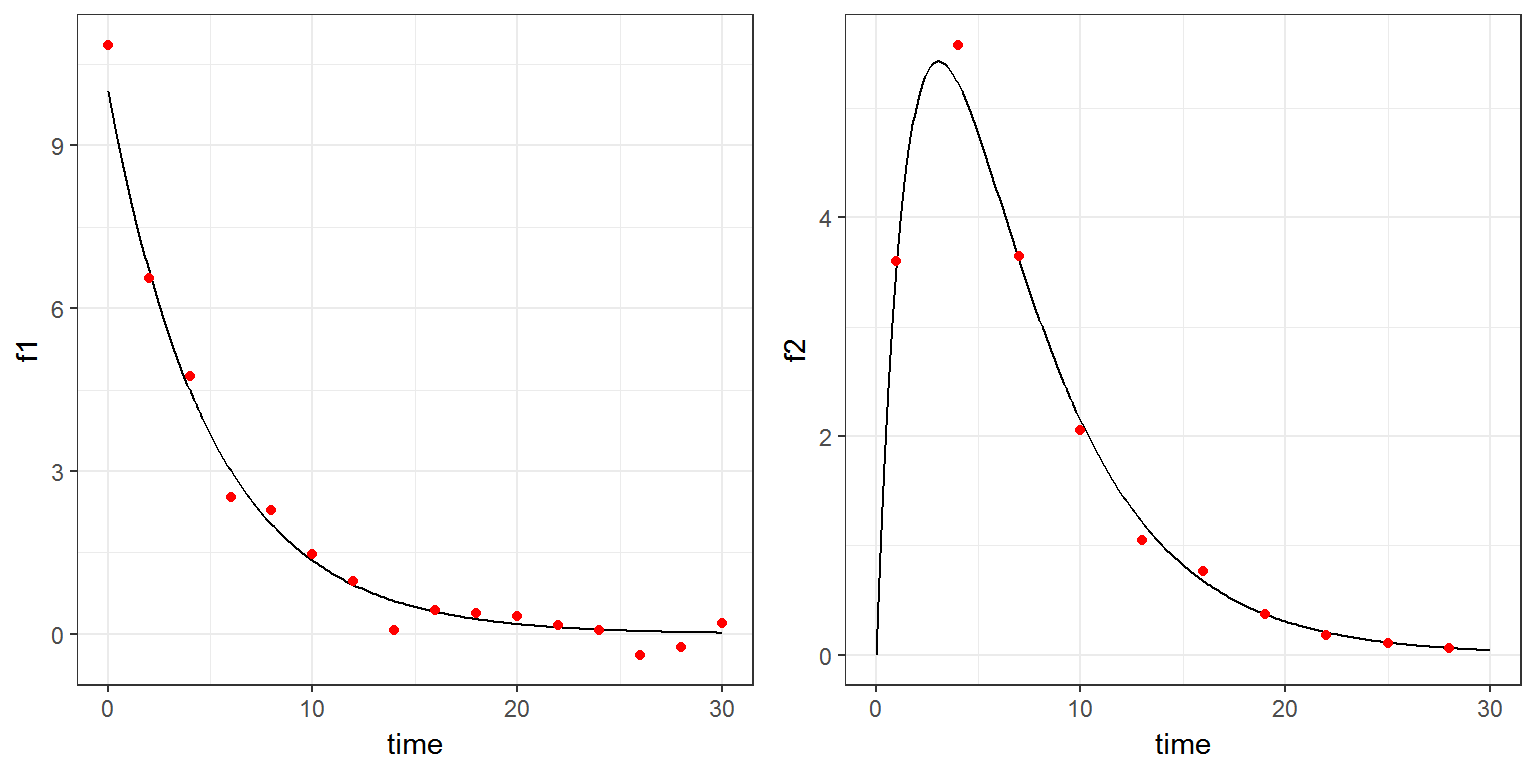R script: continuous.R

Mlxtran code: model/continuous.txt

$$\newcommand{\esp}{\mathbb{E}\left(#1\right)} \newcommand{\var}{\mbox{Var}\left(#1\right)} \newcommand{\deriv}{\dot{#1}(t)} \newcommand{\prob}{ \mathbb{P}\!(#1)} \newcommand{\eqdef}{\mathop{=}\limits^{\mathrm{def}}} \newcommand{\by}{\boldsymbol{y}} \newcommand{\bc}{\boldsymbol{c}} \newcommand{\bpsi}{\boldsymbol{\psi}} \def\pmacro{\texttt{p}} \def\like{{\cal L}} \def\llike{{\cal LL}} \def\logit{{\rm logit}} \def\probit{{\rm probit}} \def\one{{\rm 1\!I}} \def\iid{\mathop{\sim}_{\rm i.i.d.}} \def\simh0{\mathop{\sim}_{H_0}} \def\df{\texttt{df}} \def\res{e} \def\xomega{x} \newcommand{\argmin}{{\rm arg}\min_{#1}} \newcommand{\argmax}{{\rm arg}\max_{#1}} \newcommand{\Rset}{\mbox{\mathbb{R}}} \def\param{\theta} \def\setparam{\Theta} \def\xnew{x_{\rm new}} \def\fnew{f_{\rm new}} \def\ynew{y_{\rm new}} \def\nnew{n_{\rm new}} \def\enew{e_{\rm new}} \def\Xnew{X_{\rm new}} \def\hfnew{\widehat{\fnew}} \def\degree{m} \def\nbeta{d} \newcommand{\limite}{\mathop{\longrightarrow}\limits_{#1}} \def\ka{k{a}} \def\ska{k{\scriptscriptstyle a}} \def\kel{k{e}} \def\skel{k{\scriptscriptstyle e}} \def\cl{C{\small l}} \def\Tlag{T\hspace{-0.1em}{lag}} \def\sTlag{T\hspace{-0.07em}{\scriptscriptstyle lag}} \def\Tk{T\hspace{-0.1em}{k0}} \def\sTk{T\hspace{-0.07em}{\scriptscriptstyle k0}} \def\thalf{t{1/2}} \newcommand{\Dphi}{\partial_\pphi #1} \def\asigma{a} \def\pphi{\psi} \newcommand{\stheta}{{\theta^\star}} \newcommand{\htheta}{{\widehat{\theta}}}$$

# 1 Introduction

A model for longitudinal data is implemented in the section [LONGITUDINAL] of a script Mlxtran.

The probability distribution of longitudinal data is defined in a block DEFINITION, using functions of time defined in the EQUATION block.

Possible distributions for continuous data are: normal, log-normal, probit-normal and logit-normal.

Thus, if $$h$$ is one of these transformation (identity, log, probit or logit), the observations $$(y_j)$$ are defined as:

$h(y_j) = h(f(t_j ; \psi)) + g(t_j ; \psi)\varepsilon_j$

where $$f$$ and $$g$$ are defined in the EQUATION block and where $$\varepsilon_j \iid {\cal N}(0,1)$$.

Here, $$f(t_j ; \psi)$$ is the prediction of $$y_j$$.

# 2 Example

The distributions of two types of continuous data are defined in the following example: $$(y^{(1)}_j)$$ are normally distributed while $$(y^{(2)}_j)$$ are log-normally distributed:

\begin{align} y^{(1)}_j &= f_1(t_{1j}) \ + \ (a+bf_1(t_{1j}))\varepsilon^{(1)}_j \\ \log(y^{(2)}_j) &= \log(f_2(t_{2j})) \ + \ b\, \varepsilon^{(1)}_j \end{align} where \begin{align} f_1(t) &= \frac{D}{V}e^{-k\, t} \\ f_2(t) &= \frac{D\, k_a}{V(k_a - k)}(e^{-k\, t} - e^{-k_a \, t}) \end{align} and where $$D=100$$ is given.

Note that the observation times $$(t_{1j}, 1 \leq j \leq n_1)$$ for $$y^{(1)}$$ and $$(t_{2j}, 1 \leq j \leq n_2)$$ for $$y^{(2)}$$ can be different.

This model is implemented in the file model/continuous.txt:

[LONGITUDINAL]
input = {ka, V, k, a, b}

EQUATION:
D=100
f1 = D/V*exp(-k*t)
f2 = D*ka/(V*(ka-k))*(exp(-k*t) - exp(-ka*t))
g1=a+b*f1

DEFINITION:
y1 = {distribution=normal, prediction=f1, sd=g1}
y2 = {distribution=logNormal, prediction=f2, sd=b}


We will use simulx for computing both $$f_1$$ and $$f_2$$ every 0.1h between 0h and 30h, for generating observations $$y^{(1)}$$ every 2 hours between 0h and 30h and for generating observations $$y^{(2)}$$ every 3 hours between 1h and 30h

p <- c(ka=0.5, V=10, k=0.2, a=0.3, b=0.1)

f <- list(name=c('f1','f2'), time=seq(0, 30, by=0.1))

y1 <- list(name='y1', time=seq(0, 30, by=2))
y2 <- list(name='y2', time=seq(1, 30, by=3))

res <- simulx(model     = 'model/continuous.txt',
parameter = p,
output    = list(f, y1, y2))

Here, resis a list with 4 elements: f1, f2, y1 and y2 that we can display on 2 separate plots:

library(gridExtra)
plot1=ggplot(data=res$f1, aes(x=time, y=f1)) + geom_line(size=0.5) + geom_point(data=res$y1, aes(x=time, y=y1), colour="red")

plot2=ggplot(data=res$f2, aes(x=time, y=f2)) + geom_line(size=0.5) + geom_point(data=res$y2, aes(x=time, y=y2), colour="red")

grid.arrange(plot1, plot2, ncol=2)